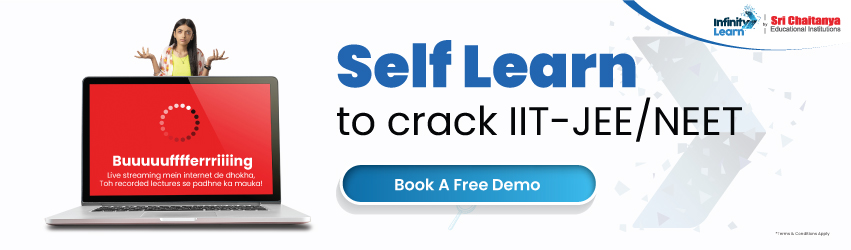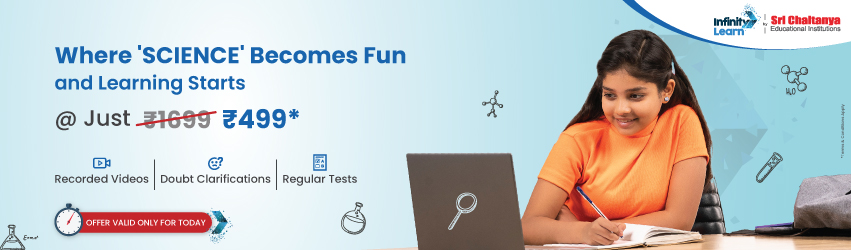CBSE Sample Papers – Class 10 Maths SA2 Solved 2016 Set 8

# CBSE Sample Papers – Class 10 Maths SA2 Solved 2016 Set 8infinitylearn surge self learn
infinitylearn starter package

## CBSE Sample Papers – Maths

Get CBSE Sample Papers for Class 10 Maths on Infinity Learn for free.

Need FREE NCERT/CBSE/IIT-JEE/NEET Study Material?

### Section A

1. Without determining the roots, comment upon the nature of the roots of the following quadratic equation:

7x2 – 6x + 1 = 03. In the given figure, if O is the centre of a circle. AB is a chord and the tangent AC at A makes an angle of 65° with AB, then find Angle AOB.4.A letter of English alphabet is chosen at random. Find the probability that the letter is a consonant.

### Section B

5. Reciprocal of a number when subtracted from the number equals to -24/5 . Find the number.

6.6th term of the Arithmetic Progression is zero. Prove that 21st term is triple of 11th term.

7. In the given figure, PT is a tangent to the circle with centre O. If QR = 6 cm, PR = 8 cm and

Triangle PRQ ~ Triangle TPO, OT/ PT8. Prove that the line segment joining the points of contact of two parallel tangents to a circle is a diameter of the circle.

9. If (x, y) is equidistant from (7, -2) and (3, 1) express x in terms of y.

10. The length of line segment joining the points A(2, -3) and B(10, y) is 10 units. If A and B are in same quadrant, find the value of y.

### Section C

11. Find the values of p so that the quadratic equation: x2 – 2p(3x – 7) – 2x + 21 = 0 has equal roots.

12. The sum of three numbers in AP is 3 and their product is -35. Find the numbers.

13. An aeroplane when flying at a height of 6 km from the ground passes vertically above another plane at an instant when the angle of elevation of the two planes from the same point on the ground are 60° and 30° respectively. Find the vertical distance between the two aeroplanes at that instant.

14. The inner circumference of a circular track is 880 m and the track is 28 m wide. Calculate the cost of levelling the track at the rate of 50 paise per square metre. Also find the cost of fencing the outer circle at the rate of Rs 10 per metre. (Take, pie= 22/7) .

15. The interior of a building is in the form of a right circular cylinder of radius 7 m and height 6 m surmounted
by a right circular cone of the same radius and of vertical angle 60°. Find the cost of painting the building
from inside at the rate of Rs 30 per m2 . (Take, pie = 22/7)

16.An iron pillar has some part in the form of a right circular cylinder and the remaining in the form of a right circular cone. The radius of the base of each cone and cylinder is 16 cm. The cylindrical part is 480 cm high and the conical part is 72 cm high. Find the weight of the pillar if 1 cubic cm of iron weigh 7.5 g.

17. Find the number of bricks, each measuring 25 cm x 12.5 cm x 7.5 cm required to construct a wall 12 m long
10 m high and 0.5 m thick, while the cement and sand mixture occupies 1/20 of the volume of the wall.

18. The internal radii of the ends of a bucket full of milk and of internal height 32 cm are 28 cm and 14 cm. If this milk is poured into a hemispherical vessel, the vessel is completely filled. Find the internal diameter of the hemispherical vessel.

19.If the points A(l, -2), B(2, 3), C(x, 2) and D(-4, -3) form a parallelogram, find the value of x and height of parallelogram taking BC as base.

20.A bag contains 20 red balls and x blue balls. If one ball is drawn from the bag, what is the probability that it is
(i) red? (ii) blue?
If the Probability of drawing red balls is 1/3 the probability of drawing blue balls determine the value of x.

### Section D22. A housewife used to spend Rs. 120 per month on the purchase of wheat at the prevailing price. When the price rose by Rs 2 per kg she found that for the same amount she could purchase 2 kg less. Find the new price per kg of the wheat.

23. Malini gets pocket money from her father everyday. Out of the pocket money, she saves Rs 3 on first day and on each succeeding day she increases her saving by 50 paise. At the end of every month, Malini purchases some books, pens and notebooks from the amount that she saved and distribute these items to needy students in her school.
(i) Find the amount saved by Malini on 10th day.
(ii) Find the total amount saved by Malini in 30 days.
(iii) What values are depicted by the act of Malini?

24.A boy standing on a horizontal plane finds a kite flying at a distance of 100 m from him at an elevation of 30°. A girl standing on the roof of 20 m high building finds the angle of elevation of the same kite to be 45°. Both the boys and girls are on opposite side of the kite. Find the distance of the kite from the girl.

25.Prove that opposite sides of a quadrilateral circumscribing a circle subtend supplementary angles at the centre of the circle.

26.In the given figure, PT is a tangent and PAB is a secant to a circle with centre O. ON is perpendicular to chord AB. Prove that:
(0 PA • PB = PN2 – AN2 (ii) PN2 – AN2 = OP2 – OT227.Draw two concentric circles of radius 3 cm and 6 cm. Taking a point on the outer circle, construct the pair of tangents to the other circle.

28.In each corner of a triangular field a horse is tethered with a rope of length 7 m. Sides of the triangle are 60 m, 80 m and 100 m.
(i) Find the area of the field over which horses can graze.
(ii) Area of the field which can not be grazed by the horses.

29.A farmer connects a pipe of internal diameter 20 cm from a canal into a cylindrical tank in his field, which is 10 m in diameter and 2 m deep. If water flows through the pipe at the rate of 6 km/h, in how much time will the tank be filled?

30.If two opposite vertices of a square are (5, 4) and (1, -6) then find the coordinates of its remaining two vertices.

31.Two dice are thrown together. Find the probability that the product of the numbers on the top of two dice is
(i) prime number, (ii) perfect square, (iii) multiple of 4, (iv) more than 36.

Join Infinity Learn Regular Class Programme!